Next: Electron spin Up: Angular momentum and its Previous: Angular momentum of coupled

#### Normal Zeeman effect

In an experiment performed by the Dutch physicist Peter Zeeman in 1896, it was observed that each spectral line in the excitation spectrum for an atom placed in a magnetic field split into number of additional lines. Furthermore, the difference in energy between the new lines and the original line was found to be directly proportional to the strength of the external field. This effect came to be known as Zeeman effect. It could only be explained using quantum mechanics which says that the orbital angular momentum is quantized both in magnitude and direction:

|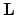| =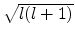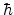and Lz = mlWe now shall try to understand the normal Zeeman effect more fully.

At first let us formulate a few classical expressions associated with an electron moving in a circular path around a nucleus as shown in the animation.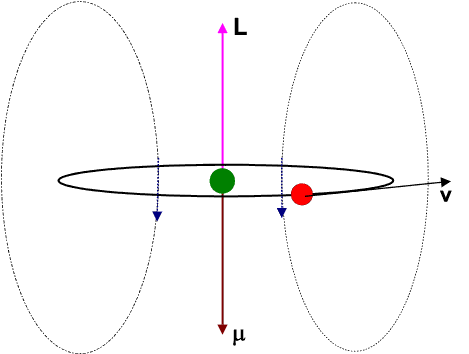The circulating electron would create a magnetic field and hence give rise to a magnetic dipole moment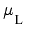. It is left for the students to derive the relation betwenand the orbital angular momentum () of the electron: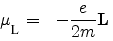Now if the current loop is placed in an external manetic field (along the z direction (say)), then the torque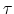on the magnetic dipole is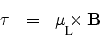and the coresponding potential energy is given by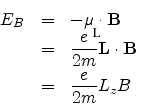According to quantum mechanics Lz = ml. Therefore the total energy isE0 is the energy in the absence of the field and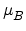=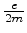is called the Bohr magneton, having dimensions the same as the dipole moment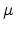.

Thus each energy level splits into 2l + 1 levels, since ml=-l to +l in integral steps.

The emission spectrum of the atom placed in the external magnetic field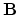will however show only two additional lines apart from the original line. This is because the selection rules for electric dipole transitions are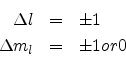The frequency or wavelength of the three lines can be obtained from the relations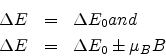The two symmetricaly placed lines on either side of the zero-field line are shifted in energy byB. These three lines constitute what is known as a triplet.

The Zeeman effect may also be explained in terms of a vector model as shown in the following figure.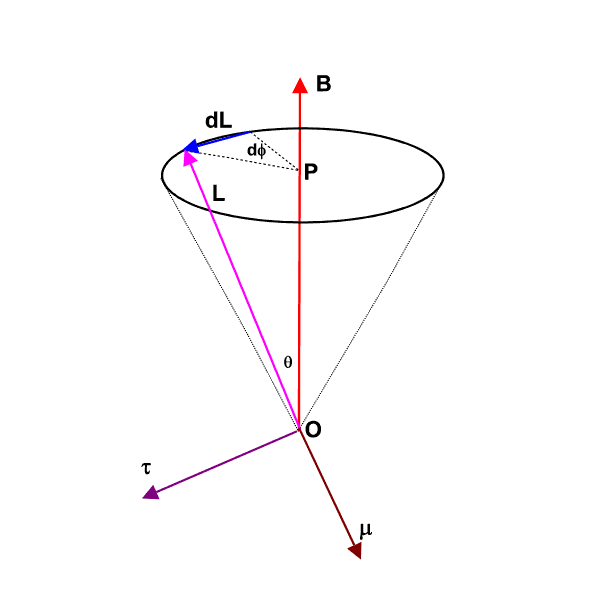The magnetic dipole momentof the electron is antiparallel to the orbital angular momentum. Both precess around the constant magnetic fieldwith an angular frequency of precession proportional to the field-strength and given by

This precession is called Larmor precession.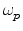is also equal to the frequency shift of the Zeeman lines originating from the external field from the field-free line. This precession of thearoundis shown in the following animation.Note that for (2l + 1) different values of ml we have (2l + 1) different values of the projection of, viz., Lz = mlor (2l + 1) different values of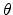. This aspect is referred to as space quantization of the orbital angular momentum in the presence of an external field.Next: Electron spin Up: Angular momentum and its Previous: Angular momentum of coupled
Abhijit Poddar
2007-09-27